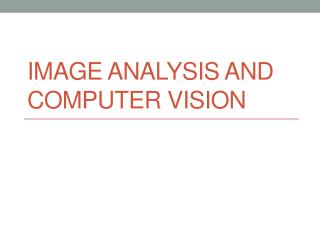DownloadDownload PresentationImage analysis and computer vision

# Image analysis and computer vision

Télécharger la présentation## Image analysis and computer vision

- - - - - - - - - - - - - - - - - - - - - - - - - - - E N D - - - - - - - - - - - - - - - - - - - - - - - - - - -
##### Presentation Transcript

1. Image analysis and computer vision

2. Image processing basic steps 1. Image Enhancement 2. Image Restoration 3. Image Analysis 4. Image compression 5. Image Synthesis

3. Goal in image analysis • Image analysis operations are used in applications, that require the measurement and classification of image information • Examples: • Cell recognition from tissue sample • Object recognition from conveyor belt • Zip code reading from envelope

4. Group discussion • List application possibilities for image analysis!

5. Image analysis • Basis is visual image, whose content should be interpreted • As a result mostly non-image data • As a goal is to understand images content classifying its content

6. Exaple: Robot vision

7. Example: Robotvehicle

8. Example: Traffic analysis

9. Image analysis operations

10. Image analysis operations • Segmentation • operation that highlights individual objects within an image. • Feature Extraction • after segmentation->measure the individual features of each object • Object Classification • classify the object to particular category segment feature classification space space space feature classification Segmentation extraction feature class image distinct objects

11. Segmentation operations • Image Preprocessing • Initial Object Discrimination • Image Morphological Operations segment feature classification space space space feature classification Segmentation extraction feature class image distinct objects

12. Image Preprocessing • In preprocessing eg. change images contrast, filter noise and remove distracting image background • Image enhancement operations is used

13. Initial Object Discrimination • Separates image objects into rough groups with like characteristics using image enhancement operations • Outlining and contrast enhancement often used

14. Initial object discrimination - example Original Binary contrast enhanced

15. Initial object discrimination - example • Sobel edge-enhancement • 1 Filter with horizontal mask • 2 Filter with vertical mask • 3 Add • -1 0 1 -1 -2 -1 • -2 0 2 0 0 0 • -1 0 1 1 2 1

16. Morphological processing • In preprocessed image the boundaries are very rough-> need to “clean up” • Morphological operations

17. Morphological operations • Binary operations • Erosion and dilation • Opening and Closing • Outlining • Skeletonization • Gray-scale operations • Top-Hat and Well transformations • Morphological gradient • Watershed edge detection

18. Binary morphology • Focus on two brightness values. black=0, white=255 • Technically same as spatial convolution • combines pixel brightness with a structuring element, looking for specific pattern • Array of logical values • (cut=AND, union=OR, complement=NOT)

19. Binary morphology - equation O(x,y) = 0 or 1 (predefined) if X =I (x,y) AND X0 =I (x+1,y) AND X1 =I (x+1,y-1) AND X2 =I (x,y-1) AND X3 =I (x-1,y-1) AND X4 =I (x-1,y) AND X5 =I (x-1,y+1) AND X6 =I (x,y+1) AND X7 =I (x+1,y+1) otherwise, O(x,y) = opposite state

20. Erosion • Reduces the size of the objects in relation to their background Mask 1 1 1 O(x,y) = 1 if “Hit” 1 1 1 1 1 1 = 0 if “Miss” 1 1 1

21. Example (1/2) Original Binary contrast enhanced Erosion image

22. Example (2/2) Binary contrast enhanced Eroded image

23. Dilation • Uniformly expands the size of object Mask 0 0 0 O(x,y) = 0 if “Hit” 0 0 0 = 1 if “Miss” 0 0 0

24. Example Binary contrast enhanced Dilated image

25. Opening • Erosion then Dilation • Removes one pixel mistakes like erosion • Object size remains Binary contrast enhanced Erosion Dilation

26. Closing • Dilation + erosion • Fills pixel wide holes • Object size remains Binary contrast enhanced Dilation Erosion

27. Cleaning Original binary contrast enhanced image Opening Cleaned = Opened and Closed

28. Outlining • Forms one-pixel-wide outlines and tends to be more immune to image noise than most edge enhancement operations • Implementation: • Eroded image subtract from original

29. Outlining Original Binary contrast enhanced

30. Outlining Eroded image Original - Eroded image

31. Skeletonizing • Make “wireframe” model from image • Uses different erosion masks • Analogy: fire, which burns object from each side

32. Gray-scale operations • Used when binary operations degrade an image • Gray-scale operation can be followed binary operation • Mask terms -255 ... 255 or “Don’t care”

33. Erosion and Dilation • Erosion reduces the size of objects by darken the bright areas in image • Dilation is inverse operation

34. Original Erosion Dilation Erosion and Dilation example

35. Opening and Closing • Opening = erosion + dilation • Opening reduces noise pixels • Closing = dilation + erosion • Closing fills one-pixel-wide holes

36. Opening example

37. Opening - example 2

38. Eroded Dilated Morphological gradient • Images outlines as a result • Make copy from image. Erosion to other image and dilation to other. Then images subtract from each other using a dual-image point process. Gradient Image Original Eroded - dilated =